# Mercury's Perihelion Prequel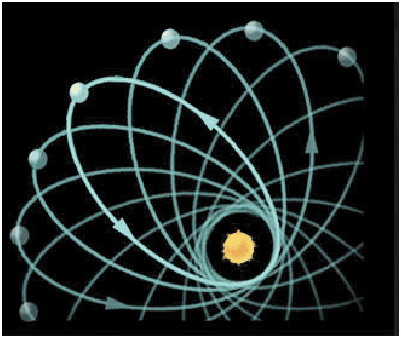Mercury's Perihelion Prequel

I had to figure out what was wrong with Paul Gerber's derivation. Gerber's work has been dismissed by the Wikipedia people and I couldn't figure out from reading their posting if the problem was in the derivation of the Gravitational Potential Formula or afterwards.

Here I derived the Precession Rate formula starting from mine (and Gerber's) Hypergeometrical Gravitational Potential. I was able to obtain the accepted formula.

Paul Gerber's work has been dismissed because he didn't have a great argument to support his potential. That is not the case any longer. My theory is presented here in details and contains all the logical support for the Hypergeometrical Gravitational Equation. I also provided the correct derivation of the Gravitational Lensing using this potential.

Currently, the mindset is such that even when a theory predicts the experimental results, it is still considered at fault because it did not produced non-observable differences based upon General Relativity equations.

Of course, this is a non-scientific reasoning. My theory predicts observed phenomena (e.g. jets comig from Black Holes - White Orifices) that are not predicted by ad-hoc Einstein equations using an ad-hoc Schwarzschild metric.

------------------------------------------------------------------------

A little research on the subject showed me that the precession was already known by the time of the prediction and was in agreement with many models where the proposers guessed left and right the actual formula for the Gravitational Potential. Some of those models had velocity dependence, although not in the manner I proposed.

In addition, the precession of Mercury was the result of many interactions with the planets of the Solar System. The unexplained precession component was of the order of 43 arc seconds per century.

Let's see if one can solve that problem in a different paradigm, say, in the Hypergeometrical Universe paradigm.

First let's write the equation for the Hypergeometrical Gravitational Force.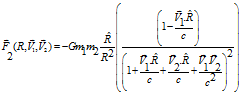(1)

Remember that this equation is not guessed (Ansatz) but derived from first principles!!!!!!!!!!!!!!!!!!!!

If we consider that the Sun is not rotating, thus V1=0 the final force sensed by Mercury is given by: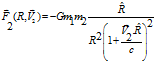(2)

Adiabatic integration upon R yields the potential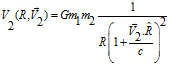(3)

Which is equation (1) on the Gerber Model presented in Jaume Gine paper.

The Kinetic Energy T per unit mass is given by: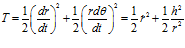Where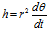Is the angular momentum per unit mass.

The Potential Energy per unit mass is given by: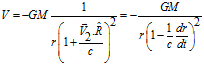The generalized momentum per unit mass is given by: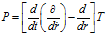Similarly the generalized force per unit mass is given by: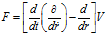Solving for P one obtains: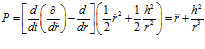Similarly for F one obtains: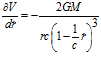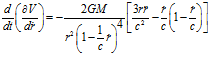Thus F becomes:Expanding it in powers of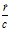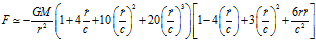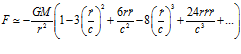Neglecting terms above the second order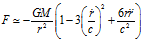Equating generalized momentum and forces yield the generalized equation of motion: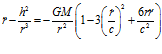Since the Gravitational force is radial, there is no tangential acceleration. Hence, integrating equation (5)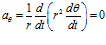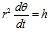Thus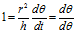Yielding the following change of variables (t->θ) and (r=1/u)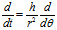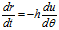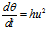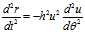thus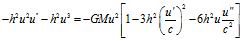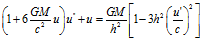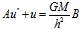This simple equation can be solved promptly if one considers that the terms in parentheses are very close to one for astronomical conditions.

The solution is given by: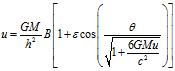Where ε is a constant of integration.

Or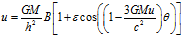The precession of Mercury's perihelion is given by the change in angle for θ=2π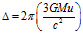If one consider that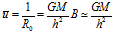Considering that the precession of the perihelion or apogee are the same, let's consider the apogee. The precession is given by: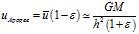Then the formula for the precession rate is given by: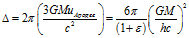Gerber and Einstein obtained a more complex version of this formula. Let's see if one can simplify Einstein's equation into ours.

Einstein equation is given by: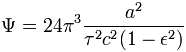ε=Eccentricity, a=Semi-major axis, τ=Orbital period.

Under standard assumptions the orbital period (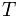) of a body traveling along an elliptic orbit can be computed as: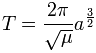where:

•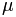is standard gravitational parameter,
•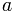is length of semi-major axis.
Replacing the period and the semi-major axis in terms of R0 yields: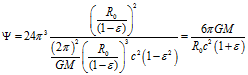Substituting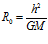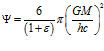Which is the same result!!!!!!!!!!!!!!!

This means that given that Gerber's Gravitational potential is also the Hypergeometrical Gravitational Potential and that Gerber's work has been totally dismissed, I cocluded that the cause was inconsistencies in the logic used to derive the Gravitational Potential.

In Gerber's Gravity, the author emphasized that despite Gerber's result yielded the observed precession, it does not provide the same equations as General Relativity – as if this were necessarily a problem.

 The gravitational radius of the Sun is m = 1.475 km and the semilatus rectum of Mercury's orbit is L = (5.544)107 km, so the precession of Mercury's orbit due to this effect (excluding the perturbations of the other planets, etc.) is 0.1034 arc seconds per revolution. Mercury completes 414.93 revolutions per century, so its orbit precesses (due to this effect) by 42.9 arc seconds per century, in excellent agreement with what is observed. This formula also gives values for all other known bodies orbiting the Sun consistent with observation. We should mention that although general relativity and Gerber's potential predict the same first-order precession (for weak fields), the respective equations of motion are not identical, even at the first non-Newtonian level of approximation. In terms of the parameter u = 1/r the equations of motion are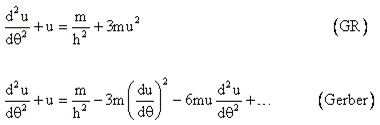so the non-Newtonian terms are actually quite different. Of course, any non-Newtonian terms will lead to orbits that fail to close, so there will be some cumulative precession for the two-body problem. It just so happens that the term +3mu2 in the GR equation of motion and the term 6mu d2u/d2 in Gerber's equation of motion both result in a first-order precession of 6m/L in the slow weak-field limit. Thus Gerber did not in any way anticipate the two-body equation of motion predicted by general relativity, let alone the field equations from which the relativistic equation of motion is derived. Nevertheless, we have verified that Gerber's potential (1) does indeed yield the observed non-Newtonian precession for planetary orbits, so we return to the question of the how Gerber arrived at this particular form, and whether it has any consistent representation.

Eventually, the author tries to derive Gerber's potential using an argument based upon retarded potentials.

Since we arrived at the same equation obtained by Gerber and Einstein and we know that there has been extensive testing of this equation on the calculation of precession of other planets, spacecrafts, etc. there is no need to pose any more arguments to support the Hypergeometrical Gravitational Equation.

Overzealous Wikipedia editors concluded that because Gerber's argument leading to the Hypergeometrical Gravitational Potential was flawed and thus he did not preceded Einstein in deriving the correct formula for the precession of Mercury's Perihelion. Currently this subtle details is very relevant because the editors believe that General Relativity equations and reality are the same. Somehow, they see other theories as ad-hoc but failed to see how ad-hoc General Relativity and Einstein equations are. Anything that describes the Universe with a parametrized (Unknon) metric cannot be anything other than an Ad-Hoc theory...:)

It also seems that Gerber made a mistake in deriving the Gravitational Lensing angle. Somehow "experts" did not catch his mistake.

I did it correctly and reached the appropriate result.
So Gehrcke initiated a reprint of Gerber's 1902-paper in the Annalen der Physik in 1917, where he questioned the priority of Einstein and tried to prove a possible Plagiarism by him.[A 5]However, according to Albrecht Fölsing[B 5] and Roseveare,[B 6] those claims were rejected, because soon after Gerber's paper was reprinted, scientists like Hugo von Seeliger,[A 6] Max von Laue[A 7] published some papers, where it was shown that Gerber's theory is inconsistent and his formula is not the consequence of his premises. Also Roseveare argued that Gerber's theory is inconsistent and that the value for the deflection of light in the gravitational field of the sun in Gerber's theory was too high by the factor 3/2. And Einstein wrote in 1920:[A 8]

 " Mr. Gehrcke wants to make us believe that the Perihelion shift of mercury can be explained without the theory of relativity. So there are two possibilities. Either you invent special interplanetary masses. [...] Or you rely on a work by Gerber, who already gave the right formula for the Perihelion shift of mercury before me. The experts are not only in agreement that Gerber's derivation is wrong through and through, but the formula cannot be obtained as a consequence of the main assumption made by Gerber. Mr. Gerber's work is therefore completely useless, an unsuccessful and erroneous theoretical attempt. I maintain that the theory of general relativity has provided the first real explanation of the perihelion motion of Mercury. I have not mentioned the work by Gerber originally, because I did not know it when I wrote my work on the perihelion motion of Mercury; even if I had been aware of it, I would not have had any reason to mention it.[C 1]
Thus the Hypergeometrical Universe Theory also "predicts" the Precession of Mercury's Perihelion, the Gravitational Lensing angle, the White Orifice phenomena and thus equates and surpasses General Relativity in its achievement. Needless to say, it provides the reason for having the speed of light as the limit, provides an alternative model for the Gravity induced metric (spacetime) deformation.

Gerber model is a limit of my theory where one considers that body 1 is not rotating. It yields the correct value for the precession constant (42.3 arc seconds per century).

Cheers,

MP

--------------------------------------------

Gerber's Results:

Paul Gerber reached an identical Gravitational Potential and from there derived the precession of Mercury's Perihelion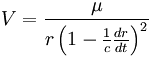According to Gerber, the relation of the speed of gravity (c) and the Perihelion shift (Ψ) is:ε=Eccentricity, a=Semi-major axis, τ=Orbital period.

It was noted by the Einstein- and relativity critic Ernst Gehrcke in 1916,[A 3] that this formula is mathematically identical to Albert Einstein's formula (1915) for general relativity.[A 4]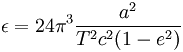, where e=Eccentricity, a=Semi-major axis, T=Orbital period.

Data on Mercury

Mercury is the closest planet to the Sun and the eighth largest. Mercury is slightly smaller in diameter than the moons Ganymede and Titan but more than twice as massive.

orbit: 57,910,000 km (0.38 AU) from Sun

diameter: 4,880 km

mass: 3.30e23 kg

Mercury's orbit is highly eccentric; at perihelion it is only 46 million km from the Sun but at aphelion it is 70 million. The position of the perihelion precesses around the Sun at a very slow rate. 19th century astronomers made very careful observations of Mercury's orbital parameters but could not adequately explain them using Newtonian mechanics. The tiny differences between the observed and predicted values were a minor but nagging problem for many decades. It was thought that another planet (sometimes called Vulcan) slightly closer to the Sun than Mercury might account for the discrepancy. But despite much effort, no such planet was found. The real answer turned out to be much more dramatic: Einstein's General Theory of Relativity! Its correct prediction of the motions of Mercury was an important factor in the early acceptance of the theory.

Data on Sun

Our Sun is a normal main-sequence G2 star, one of more than 100 billion stars in our galaxy.

diameter: 1,390,000 km.

mass: 1.989e30kg

temperature: 5800 K (surface)

15,600,000 K (core)

The Sun is by far the largest object in the solar system. It contains more than 99.8% of the total mass of the Solar System (Jupiter contains most of the rest).

## Orbital period

Under standard assumptions the orbital period () of a body traveling along an elliptic orbit can be computed as:where:

•is standard gravitational parameter,
•is length of semi-major axis.

Conclusions:

• The orbital period is equal to that for a circular orbit with the orbit radius equal to the semi-major axis (),
• The orbital period does not depend on the eccentricity (See also: Kepler's third law).
Currently unrated

### New Comment

required

required (not published)

optional

required